| Home | | Advanced Mathematics |

## Chapter: Biostatistics for the Health Sciences: Nonparametric Methods

Biostatistics for the Health Sciences: Nonparametric Methods - Exercises questions answers

EXERCISES

14.1 Apply the Wilcoxon rank-sum test to the following problem; we have modified the data from the pig blood loss experiment:Do the results differ from the standard two-sample t test with pooled variance? Are the p-values similar?

14.2 Apply the Wilcoxon rank-sum test in the following case to see if schizophrenia is randomly distributed across the seasons:

Season of Birth Among 100 Schizophrenic Patients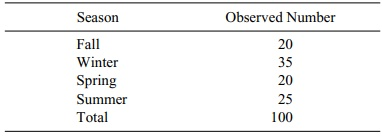14.3 Using the following modification of the city data, apply the Wilcoxon signed-rank test to determine whether there is a difference in average tem-perature between the two cities. Compare your results to a paired t test.

Daily Temperatures for Two Cities and Their Paired Differences14.4 Apply the sign test to the above example. Did the results change? Which test is more powerful, the sign test or the Wilcoxon signed-rank test? Why?

14.5 Suppose we compare four instructors for consistency of grading. Use the following table to apply the Kruskal–Wallis test to determine whether there is a difference among instructors.

Grade Counts for Students by Instructor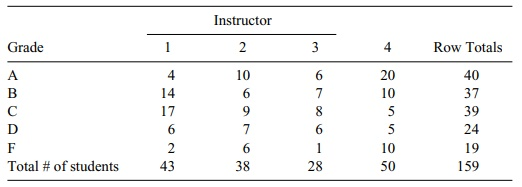14.6 Based on the temperature data in Exercise 14.3, use the day pairing to com-pute a Spearman rank order correlation between the two cities.

14.7 Use the modified aggressiveness scores for twins (given in the table below) to apply the Wilcoxon signed-rank test. What is the p-value?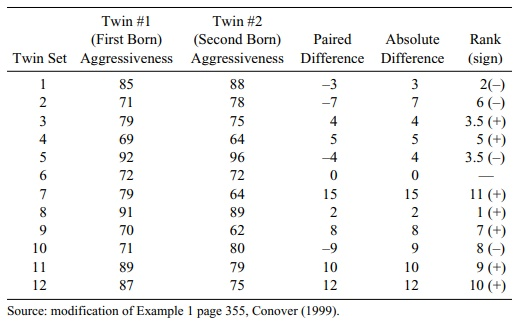14.8 Apply the sign test to the data in Exercise 14.7. Does the result change? What is the p-value?

14.9 Using the modified aggressiveness scores with the aid of the table below, determine Spearman’s rank order correlation for the twins.

Aggressiveness Scores for 12 Sets of Identical Twins14.10 Recall the Lady Tasting Tea example. Suppose that instead of being given four cups with milk poured first and four cups with tea poured first, the lady was given five cups with milk poured first and five cups with tea poured first. Suppose the outcome of the experiment was as shown in the table at the top of the next page.

Observed 2 × 2 Contingency Table for Fisher’s Exact Testa. Determine the more extreme tables.

b. Do a two-sided Fisher’s exact test at the 0.05 level of the null hypothesis that the lady is guessing randomly.

c. Do a one-sided test at the 0.05 level.

d. What is the p-value for the two-sided test?

e. What is the p-value for the one-sided test?

f. Which test makes more sense here, one-sided or two-sided?

14.1 First let us look at the two sample t test. The control group mean is 1687.4 and the treatment group mean is 1255.9. The pooled estimate of the standard deviation is 1073.075.

The t statistic is (c –t)/(Sp 2/n), where n is the sample size in each group. Since n = 10, Sp = 1073.75, and the mean difference is 614.325, t = 0.8992. This is not significant for a t with 18 degrees of freedom. Now consider the Wilcoxon test. We may see different results because the distributions are very nonnormal. Consid-er the following table: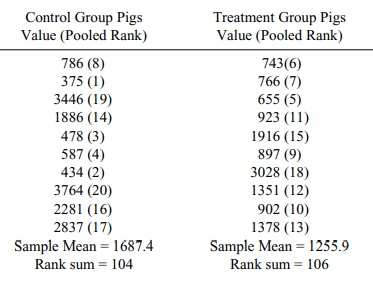The rank sum for the first sample is 104 and the rank sum for the control group and the treatment group is 106. They are virtually the same. Both tests lead to the same conclusion. The two-sided p-value for the t test is close to 0.40. For the Wilcoxon test it is 0.97 for the two-sided p-value and 0.38 for the t test (based on SAS results). The p-values are similar.

14.3 We consider the following table:

Daily Temperatures for Two Cities and Their Paired Differences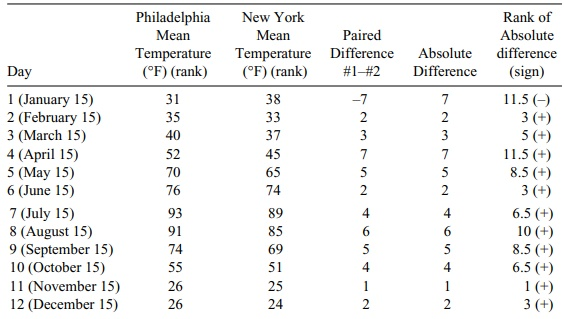For the paired t test, we have a mean difference of 2.833. The standard deviation of the differences is S = 3.589 and the t statistic is t = 2.833/1.036 = 2.734. This is a Student t with 11 degrees of freedom under the null hypothesis. For a two-sided 0.02 significance level, the critical t is 2.718. So since 2.734 > 2.718, the p-value is less than 0.02.

The sum of the negative ranks is only 11.5, whereas the sum of the positive ranks is 66.5. If the null hypothesis were true, we would expect these ranks to be approximately equal at around 39. The null hypothesis is clearly rejected in this case. We get an approximate p-value by using the normal approximation, Z = (11.5–39)/ [(2n + 1)(39)/6], where n = 12 is the number of pairs. So Z = –27.5/ [25(39)/6] = –27.5/12.75 = –2.157. This is a one-sided p-value of 0.5 – 0.4845 = 0.0155 or two-sided p = 0.031. This agrees closely with the result for the paired t test.

14.9 We use the following table:

Aggressiveness Scores for 12 Identical TwinsRecall that the rank correlation is given by the following formula: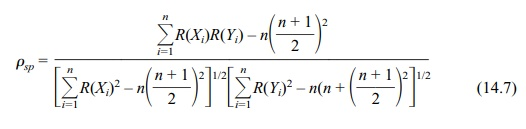where n is the number of ranked pairs, R(Xi) is the rank of Xi, and R(Yi) is the rank of Yi.

The numerator is 612.5 – 12 (13/2)2 = 612.5 – 507 = 105.5. The terms in the denominator are (649 – 507)1/2 and (649 – 507)1/2. So ρsp = 105.5/(649 – 507) = 105.5/142 = 0.743 a strong positive relationship.